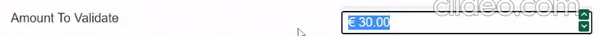# Numeric validation flaw with decimal

Hello @Team

I couldn't find an example in the demos, so I'm posting my case.
The Razden numeric only validates out of range one time and updates the field to the max value.
Then all invalid values would be accepted. Writing a correct value resets this behavior.``````<RadzenNumeric TValue="decimal" @bind-Value=@(value) Format="@(\$"{CurrencySymbol} 0.00")" Min="0.01m" Max="30.0m" Step="0.50m"/>
``````

The only option I can offer to avoid this is to use Change event instead @bind-Value:

``````<RadzenNumeric TValue="decimal" Min="0.01m" Max="30.0m" Step="0.50m" Change=@(args => value = args)/>

@code {
decimal value;
}
``````

Thank you. That helped.